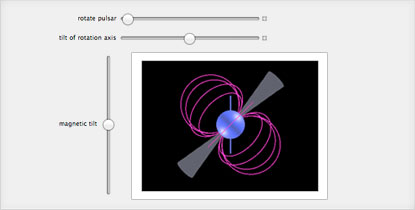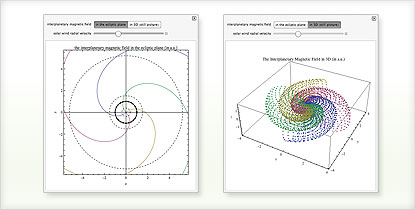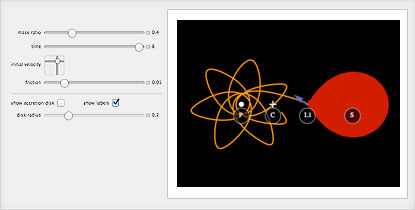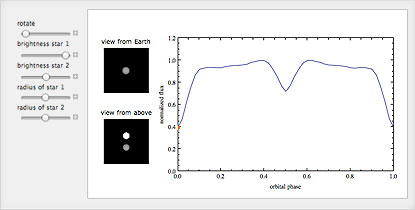# The Wolfram Solution for Astronomy

Compute positions of astronomical objects in real time, building models, analyzing data and instantly creating interactive visualizations—all in one system with one integrated workflow.

The Wolfram astronomy solution combines sophisticated data analysis capabilities, world-class image processing and highly optimized differential equation solving with built-in parallel computing and 64-bit technology.

• The Wolfram Edge
• How Wolfram Compares
• Key Capabilities

Wolfram technologies include thousands of built-in functions and curated data on many topics that let you:

• Do mathematical modeling to study galaxy formation, stellar dynamics and other topics
• Perform image processing of astronomical objects
• Use built-in astronomy data for real-time computation of positions of astronomical objects
• Perform sophisticated data analysis and optimization routines for astrophysical modeling
• Plot orbits of planets, exoplanets, stars and other astronomical bodies using built-in astronomical data
• Perform spectral analysis of irregularly sampled time-series data
• Model particle trajectories
• Compute the rise times of stars and planets through the yearModeling and interactively exploring a pulsarPlotting the interplanetary magnetic field (Parker spiral)

Does your current tool set have these advantages?

• Free-form linguistic input produces immediate results without the need for syntax
Unique to Wolfram technologies
• Built-in astronomy data for real-time dynamic computation of astronomical object positions
Unique to Wolfram technologies
• Automatic interface construction to interactively visualize your simulations, examine model sensitivity to parameter changes and more
Unique to Wolfram technologies
• Tight integration of the core Mathematica system with more than 20 built-in application areas such as image processing, GIS, statistics and more
Matlab requires multiple extra-cost toolboxes
• Powerful symbolic statistical computation and data analysis using built-in functions
R, STATA and other numerical systems do not have integrated symbolic functionality
• Fully automated precision control and arbitrary-precision arithmetic produce accurate results
All systems relying on finite precision arithmetic, such as Excel or Matlab, can cause errors due to lack of precision
• Seamless integration of numerics, symbolics, interactive graphics and all other computational aspects in one systemModeling mass transfer in binary star systemsModeling light curves of objects too far away to study with optical telescopes

# Consulting Solutions

Enlist the world’s computation experts to help with your project—any size, any level. At Wolfram, we know what’s possible with computational technology because we are global leaders in creating it. That gives us an unprecedented depth of expertise in applying it to consulting work in a variety of fields. Whether individual or enterprise, from concept to deployment, our computation experts can help you achieve robust results with less time and effort. Get us started with your project today